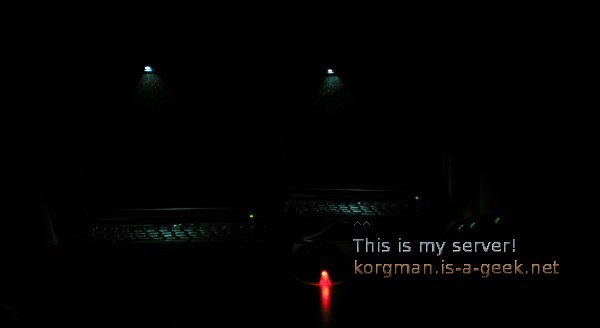# Watt at standby (ThinkPad X40 - Pentium-m)

In order to measure how much watts per hour the computer need for stand-by, I used the smart data of the battery via linux kernel.
The battery is fully charged (last value ENERGY_NOW: 620200000μW = 62Wh). I closed the computer at 00:50 2012-07-27,00:50.01,0.51,POWER_SUPPLY_ENERGY_FULL=63420000,POWER_SUPPLY_POWER_NOW=0,POWER_SUPPLY_STATUS=Unknown,POWER_SUPPLY_ENERGY_NOW=62020000

After 10 hours the watts are 58130000μW = 58 Wh. 4 watts for 10 hours of standby.

0.4 watts for 1 hour. 2012-07-27,11:00.01,0.42,POWER_SUPPLY_ENERGY_FULL=63420000,POWER_SUPPLY_POWER_NOW=13408000,POWER_SUPPLY_STATUS=Discharging,POWER_SUPPLY_ENERGY_NOW=58130000

Τυχαία εικόναt20_t21_server_back_dark_txt.jpg

You are here with: CCBot/2.0 (https://commoncrawl.org/faq/)
Made in Linux :)